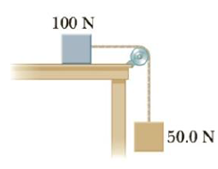Chapter 4, Problem 74AP

Chapter
Section
Textbook Problem

(a) What is the minimum force of friction required to hold the system of Figure P4.74 in equilibrium? (b) What coefficient of static friction between the 100.-N block and the table ensures equilibrium? (c) If the coefficient of kinetic friction between the 100.-N block and the table is 0.250, what hanging weight should replace the 50.0-N weight to allow the system to move at a constant speed once it is set in motion?Figure P4.74

(a)

To determine
The minimum friction force.

Explanation

Given Info: The weight of the blocks are 100 N and 50 N. The co-efficient of kinetic friction is 0.250.

For the hanging block, the tension in the rope (T) is equal to the weight of the block.

T=50N

For the upper block, the tension in the rope equal the friction force ( Fs )

(b)

To determine
The co-efficient of static friction.

(c)

To determine
The weight of the block.

Still sussing out bartleby?

Check out a sample textbook solution.

See a sample solution

The Solution to Your Study Problems

Bartleby provides explanations to thousands of textbook problems written by our experts, many with advanced degrees!

Get Started